## Confocal Ellipsoidal Coordinates

The confocal ellipsoidal coordinates (called simply ellipsoidal coordinates by Morse and Feshbach 1953) are given by the equations(1)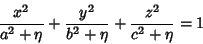(2)(3)

where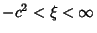,, and. Surfaces of constant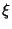are confocal Ellipsoids, surfaces of constantare one-sheeted Hyperboloids, and surfaces of constant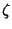are two-sheeted Hyperboloids. For every, there is a unique set of ellipsoidal coordinates. However,specifies eight points symmetrically located in octants. Solving for,, andgives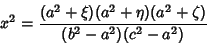(4)(5)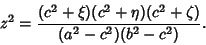(6)

The Laplacian is(7)
where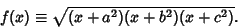(8)

Another definition is(9)(10)(11)

where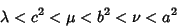(12)

(Arfken 1970, pp. 117-118). Byerly (1959, p. 251) uses a slightly different definition in which the Greek variables are replaced by their squares, and. Equation (9) represents an Ellipsoid, (10) represents a one-sheeted Hyperboloid, and (11) represents a two-sheeted Hyperboloid. In terms of Cartesian Coordinates,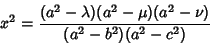(13)(14)(15)

The Scale Factors are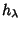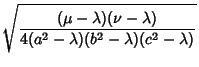(16)(17)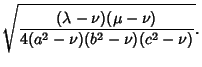(18)

The Laplacian is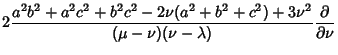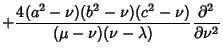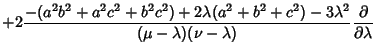(19)

Using the Notation of Byerly (1959, pp. 252-253), this can be reduced to(20)

where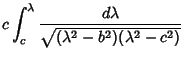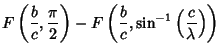(21)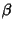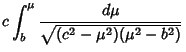(22)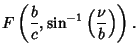(23)

Here,is an Elliptic Integral of the First Kind. In terms of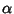,, and,(24)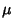(25)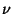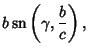(26)

where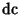,andare Jacobi Elliptic Functions. The Helmholtz Differential Equation is separable in confocal ellipsoidal coordinates.

References

Abramowitz, M. and Stegun, C. A. (Eds.). Definition of Elliptical Coordinates.'' §21.1 in Handbook of Mathematical Functions with Formulas, Graphs, and Mathematical Tables, 9th printing. New York: Dover, p. 752, 1972.

Arfken, G. Confocal Ellipsoidal Coordinates.'' §2.15 in Mathematical Methods for Physicists, 2nd ed. New York: Academic Press, pp. 117-118, 1970.

Byerly, W. E. An Elementary Treatise on Fourier's Series, and Spherical, Cylindrical, and Ellipsoidal Harmonics, with Applications to Problems in Mathematical Physics. New York: Dover, 1959.

Morse, P. M. and Feshbach, H. Methods of Theoretical Physics, Part I. New York: McGraw-Hill, p. 663, 1953.